﻿ Valorization of Freshwater Seashells (<i>Corbula</i> <i>trigona</i>) in the Aim of Nitrates Removal in Aqueous Environment: Optimization of the Treatment by the Methodology of Surface ResponsesAmerican Journal of Chemistry

p-ISSN: 2165-8749    e-ISSN: 2165-8781

2022;  12(2): 40-50

doi:10.5923/j.chemistry.20221202.03

Received: Mar. 28, 2022; Accepted: Apr. 30, 2022; Published: May 12, 2022### Valorization of Freshwater Seashells (Corbulatrigona) in the Aim of Nitrates Removal in Aqueous Environment: Optimization of the Treatment by the Methodology of Surface Responses

Dan Nixon Nonh1, Sadat Aw1, Sorho Siaka1, Noureddine Bousserrhine2

1Laboratoire des Procédés Industriels, de Synthèse, de l’Environnement et des Energies Nouvelles (LAPISEN), Institut Nationale Polytechnique Félix Houphouët Boigny (Côte d’Ivoire), Yamoussoukro, Côte d’Ivoire

2Laboratoire Eau, Environnement Systèmes Urbains, Université Paris Est Créteil (UPEC). Faculté des Sciences et Technologie, LEESU, 61, Avenue Général De Gaulle, CRETEIL

Correspondence to: Dan Nixon Nonh, Laboratoire des Procédés Industriels, de Synthèse, de l’Environnement et des Energies Nouvelles (LAPISEN), Institut Nationale Polytechnique Félix Houphouët Boigny (Côte d’Ivoire), Yamoussoukro, Côte d’Ivoire.
 Email: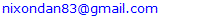Abstract

This study was about the removal of nitrates in synthetic solutions in batch mode. It put into contribution the use of freshwater seashells (Corbula trigona) as adsorbents. Corbula trigona Adsorbents (ACT) have been characterized by means of analytical techniques such as X-Ray Fluorescence (XRF), Fourier Transform Infrared (FTIR), scanning electron microscopy coupled with the EDX and the X-Ray Diffraction (XRD). This characterization allowed to highlight the elemental composition, the predominant functional groups, the morphology of their surface and to identify the crystal phases. The effects of the different variables which influence the process of the nitrate removal have been studied thanks to a Placket-Burman Design. The concentration of the pollutant, the middle pH and the absorbent mass were the parameters which influenced the studied response. It comes out that the influence of those parameters on the nitrate removal can be respectively rated at 66.16%, 27.05% and 4.21%. The results analysis show that the absorbent mass has a negative impact whereas the pollutant concentration and the middle pH have positively impact the effectiveness. After optimizing the process with the help of the Box-Behnken Design (BBD), the optimum conditions for a removal at 53.76%, of water containing 30mg/L of nitrates, one needs 1.11 g of a pH 2 material. The corbula trigona seashells could be promising in the removal of nitrates and the Box-Behnken Design was the most effective in designing the process and determining the optimum conditions.

Keywords: Adsorption, Removal, Corbula trigona, Nitrate, Optimization, Water

Cite this paper: Dan Nixon Nonh, Sadat Aw, Sorho Siaka, Noureddine Bousserrhine, Valorization of Freshwater Seashells (Corbula trigona) in the Aim of Nitrates Removal in Aqueous Environment: Optimization of the Treatment by the Methodology of Surface Responses, American Journal of Chemistry, Vol. 12 No. 2, 2022, pp. 40-50. doi: 10.5923/j.chemistry.20221202.03.

### 2. Materials and Methods

#### 2.1. Collecting and Preparation of Corbulatrigona Adsorbent(ACT)

The seashells of the phylum Mollusk belong to the bivalves category and the Corbulinae family from the Corbula trigona have been collected by a mere collection session on the beaches of the Lagoon Aby located in the southeast of Côte d’Ivoire and sent into laboratory. Once in lab, the seashells have been softly washed and rinsed with a distilled water. After this, they were dried at a room temperature (25°C) during 3 hours, before being kept at 105°C into an incubator for 24 hours long. After they were dried, they were grind into a porcelain-maid mortar and the obtained powder has been sifted at 200 µm and 100µm . The obtained powders known ACT have been stored into glass jar till their use.

#### 2.2. Analytical Techniques for the Characterization of Corbulatrigona Adsorbent(ACT)

Several equipment have been used into the seashells characterization process and their physico-chemical properties identification. The water content has been obtained by ACT drying up into incubators at a 105°C temperature followed by a cooling into vacuum dryer. The porosity, the apparent and real density of different seashells aggregates have been determined thanks to a graduated cylinder of 100 mL. The pHpzc or pH said at zero load points, corresponds to the pH for which, the absorbents surface is null, has been determined according to the described method . In so doing, in an Erlenmeyers series of 100 mL containing 50mL of distilled water, the pH has been adjusted from 2 to 12 aid of hydrochloric acid solution or sodium at 0.1M. After that, a quantity of 50 mg of seashells sample has been added into each solution. The Erlemeyers seashells suspension materials have been maintained to agitation, to a room temperature (25°C), during 24 h. After the settling, aqueous solutions have been sifted by means of Whatman Paper of 0.45µm and the final measured pH. The pHpzc is graphically determined on a curve of pHf according to pHi. The intersection of the curve with the axis which passes by the zero corresponds to the pHpzc. The Scanning Electron Microscopy coupled with X-Ray Diffraction (XRD) (Hitashi FEG S 4800) has been used to appreciate the morphological surface of materials under a 15kV acceleration voltage. As for the elementary composition, an OXFORD-MDX1000 model of fluorescence (XRF) has been used. The powdered sample was prepared in pellet form. The obtained pellet was subjected to a source of primary X-ray radiation, after which the atoms were excited to emit secondary X-ray fluorescence characteristic of the chemical composition of the sample to be analyzed.
The X-ray DRX Rigaku Miniflex II diffraction has been used to identify the crystalline phase.
The determination of the crystalline phase was done by a Rigaku Miniflex II diffractometer (15 kV, 40 mA, Cukα: λ= 1.542 Å, scan speed of 4.min-1) for a time of 2 hours ranging from 20 and 80°C.
An Alpha Brucker FTIR spectrometer type has been used to identify the ATC functional groups between 2000 cm-1 and 500cm-1. For functional group analysis, 5 mg of finely ground shell were mixed with 300 mg of KBr. The analysis of the pellets was performed at the wave number between 2000 and 500 cm-1 with a resolution of 4 cm-1.

#### 2.3. The Preparation of the Nitrate Solution and the Adsorption Experimental Procedure

An initial synthetic solution of 100mg/L concentration has been prepared from potassium nitrate KNO3 (Panreac, purity 99%). The reactive has firstly dried into a stove at 105°C during 24 h and a bulk of 0.489 g is dissolved into distilled water to prepare a 3 L solution. From that solution, through the dilution of solutions from that 30mg/L and 60mg/L have been prepared for the removal tests. The use of one pH-meter (HANNA HI 98150), the initial pH initial of each solution has been adjusted with NaOH (1M) or HCl (1M) solutions. These 50 mL volume solutions have been shacked into a bain-marie at 25°C with at stirring speed of 220 tr/min during different moments. After sifting on a 0.45µm Whatman paper, they are analyzed with spectrophotometer (HACH DR 1900) with a wavelength of 500 nm, focusing on the AFNOR NFT 90-045 norm. In order to observe the seashells absorbent power, the removal rate of nitrate has been calculated relying on the Equation: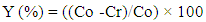(1)
With C0 and Cr which are respectively the initial and residual concentration of nitrates ions.

#### 2.4. Experimental Designs of Methodology

##### 2.4.1. Screening the Influential Factors
The nitrate removal in solution has been realized through the successively using of Plackett- Burman and Box-Behnken Design . Firstly, one of Plackett-Burman Designs has been used to set up the screening of five factors upon the residual concentrations of nitrates represented by the answer of Y. The levels (low (-1) and high (+1)) of the five factors are the following: the granulometry (100 µm - 200 µm), the adsorbent dose (0.5 g - 2 g), the time of contact (20 min-80 min), the solution concentration in nitrate (30 mg/l - 60 mg/l) and the pH of the experimental level (2 – 8). They are respectively referred to as X1, X2, X3, X4 and X5. The choice of different levels: (-1 or +1) some factors have been allotted parting from laboratory exploratory tests. The Plackett and Burman Design permits to establish a mathematical modal of first degree linking the answers to the factors. The equation of the modal to be established is displayed like the following: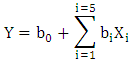(2)
Where b0 represents the mean value of the eight (8) tests answer and, bi the coefficient of the principal effect of the factor i on the answer.
The significance of the factors can be reinforced by the Pareto diagram which evaluates the different contributions expressed as follow: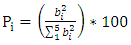(3)
Where bi is the estimate coefficient of the factor i.
The five independent factors with their levels (weak (-1), and high (+1)) are summarized on the table (1).
 Table 1. Experimental Domain of the Plackett and Burman Design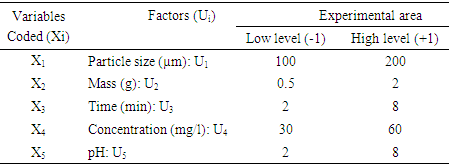##### 2.4.2. Optimization of Nitrates Removal by the Box-Behnken Design
After the screening step, three selected factors (the absorbent mass, the pollutant initial concentration and the middle pH) have been used to monitor the evolution of the nitrate residual concentration (answer) by the of Response Surface Methodology (RSM). This methodology is a collection of mathematical and statistical tools which permits to design and optimize the processes with a minimum of experience . This method also permits to establish second degree designs  of the form.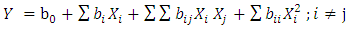(4)
Where: Xi and Xj are the independent encoded variables. bo, bi, bii and bij are the regression coefficients for the interception, respectively linear, quadratic and of interaction. The three independent variables with their three levels (weak (-1), medium (0) and high (+1)) are summed up in this table (2).
 Table 2. Experimental area of the Box-Behnken design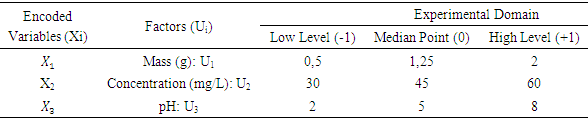Required number of experiences in elaborating the Box-Behnken design has been determined from the relation: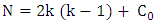(5)
Where: N the number of total experiences, k is the number of variables and C0 the number of tests at the center of the experimental domain. The conversion of real values Ui in encoded values X is provided by the relation [33-35]: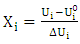(6)
Where: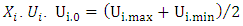et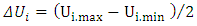respectively represent the encoded value, the real value, the median point real value of the experimental domain and the no variation of the i factor.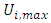and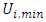respectively represent the maximal and minimal values of the real variable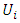. The software NEMROD-W (French 9901 Version, LPRAI-Marseille Inc., France) has been used to parse the statistical data and build response surfaces.

### 3. Results and Discussion

#### 3.1. Characterization of the Adsorbents

##### 3.1.1. Scanning Electron Microscopy Analysis Coupled with EDX
An ACT micrography obtained by SEM shows that the prepared materials are displayed under a form of particles of variable size parting from fine particles to bigger ones with a little space between them (fig. 1). This heterogeneity of the particles granulometry would probably be the consequence of the seashells and/or at the retain preparation mode. (crushing aid of a pestle in a mortar). The analysis of the EDX spectrum (fig. 2) has revealed that the seashells are principally composed of carbon (25.49%), oxygen (64.80%) and calcium (9.60%). The other elements namely the aluminum (0.07%) and the silicon (0.04%) are present at the state of trace (0.1%) as the table (3) displays. In order to refine these results and above all know under what form these elements are represented into the materials, an XRF analysis has been implemented.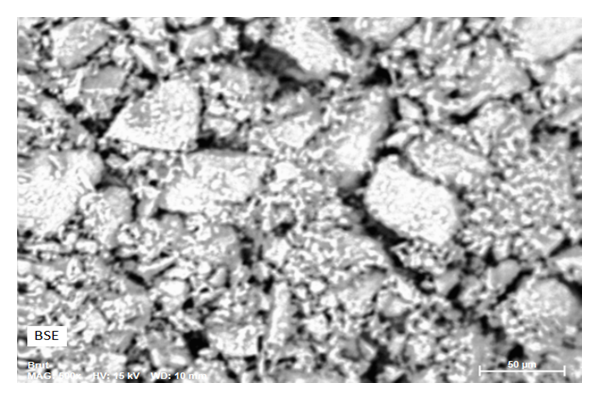Figure 1. Surface morphology of Corbula trigona adsorbents ACT obtained by SEM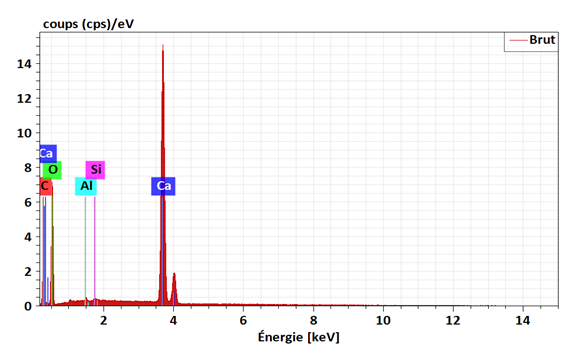Figure 2. EXD Spectrum of Corbula trigona Absorbents (ACT)
 Table 3. Elemental Chemical Composition of ACT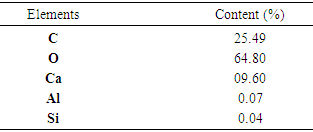##### 3.1.2. Fluorescence Analysis (XRF)
The results of the ACT XRF analysis are recapitulated in table (4).
 Table 4. Elemental Composition of ACT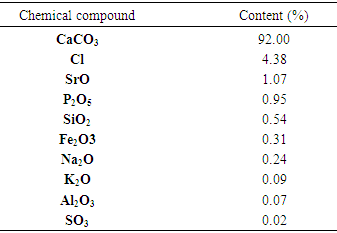From this table (4), it comes out that the calcium carbonate (CaCO3) is the principal constituent of these materials with a content of 92%. The other chemical compositions are represented under the form of trace except the Chlorine (Cl) which possesses a content more relatively abundant content (4%). These results are in accordance with the ones of  which showed that mollusk seashells are generally rich in calcium carbonate.
##### 3.1.3. Fourier Transform Infrared (FT-IR) Spectroscopy Analysis
In order to determine the functional groups, a Fourier Transform Infrared (FT-IR) Spectroscopy Analysis has been processed on the materials before and after the removal of nitrate ions. The results are displayed on figure (3).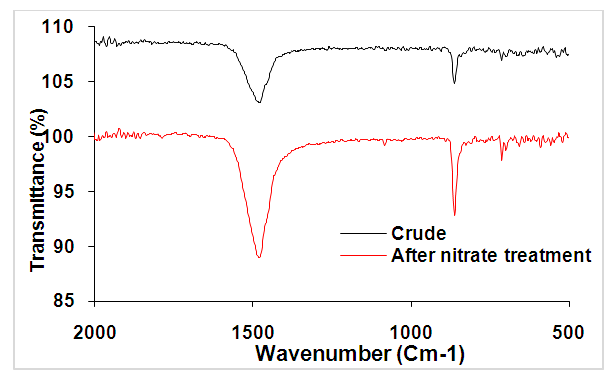Figure 3. FTIR spectrum of ACT before (curve in black) and after nitrate ion removal (curve in red)
Of these spectrums, it comes out that, the ACT, before the nitrate removal showed adsorptions bands at 861,314 and 1489,951 cm-1 corresponding to the stretched bands of the carbonate fraction . Furthermore, the bands of the aragonite can be visualized at 875 and 714 cm-1 whereas the band 1489.951 cm-1 is the calcite absorption characteristic peak . Those results show that the ACT major components are calcite and aragonite . The spectrum overlapping before and after the absorption permit to put into evidence the intensity increase of ACT characteristic peaks. This variation could be explained by the nitrate ions fixation at the ACT surface. This fact, leads to an increase of vibration of different functional groups that were initially present. In order to well appreciate those changes at the materials surface, a (XDR) analysis has been processed.
##### 3.1.4. X-Ray Diffraction Analysis
The XDR analysis permits to have a better appreciation of the effects on the the materials surface submitted to diverse actions. The variations at the materials surfaces before and after the nitrate removal have been evaluated by XRD. Figure (4) which presents an overlapping of spectrums from XRD analysis before and after the nitrates absorption show that the principal composition is the calcite observed at 2ϴ= 29,4°. Some peaks of calcite and aragonite were observed on the diffraction at 2ϴ = 20.92°; 23.76°; 26.68°; 28.32°; 33.96; 36.6°; 37.52°; 39.52°; 42.92°; 47.52°; 48. 56° . After the nitrate ions removal, the majority of the peaks on the pictogram disappeared, whereas some other peaks of a weaker intensity appeared. As a matter of fact, the interaction of nitrate ions and with the seashells during the removal process has led to the disappearance of the majority of the former peak to give way to newer ones. The XRD spectrum are in harmony with the ones of the IR represented above.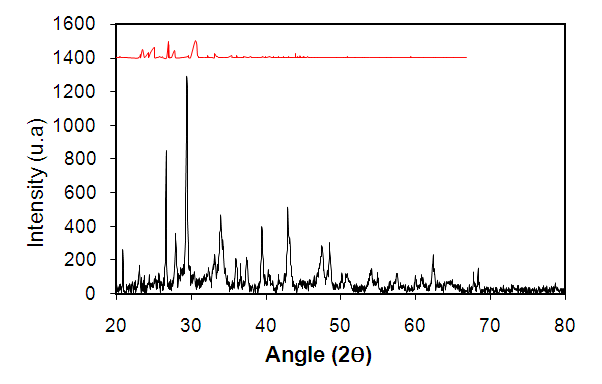Figure 4. XRD spectrum of ACT before and after nitrate ion removal (before removal in black and after removal in red)
##### 3.1.5. ATC Physico-chemical Characteristics
The results from the analysis of water content, of the apparent density (ρa) and real density (ρr), of the porosity, and the pH at zero load points (pHpzc) are indicated in the table (5).
 Table 5. Corbula trigona physico-chemical parameters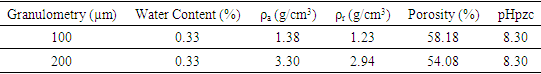Of this table, it comes out that the materials water content is relatively low because of inferior to 5%. It throws light on the free water evaporable at 100°. Additionally, one can remark that the densities apparent and real values depend on the materials granulometry. Thus, the lowest values are observed for the finest particles (100 µm). According to the literature, the volume of the absorbents used in water processing must be superior to 0,35g/cm3. Thereby, the absorbents elaborated in this way seem to be eligible for water processing. The pH at zero load points (pHpzc) being superior to 7, globally indicates the corbula trigona seashells basic character.

##### 3.2.1. Identification of Key Factors Influencing Nitrate Removal
A screening design of Plackett Burman factors has been used to identify the influential factors and their major effects on the ions nitrates removal the different results are gathered in table (6).
 Table 6. Experimental results on nitrate removal by Adsorbents Corbula trigona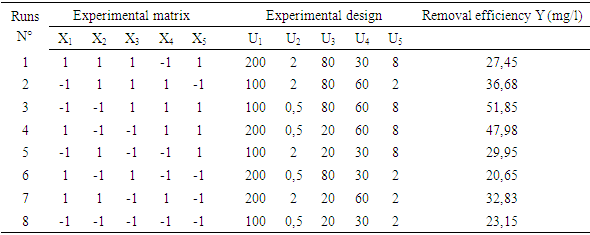Table (6) presents the results of 8 tests. After analyzing the results, one assists to a relatively important variation of the nitrates residual concentrations according to the variation of the factors level. The residual contents vary from 20.65 to 51.85 with a standards deviation of 1.828 and a determination coefficient of 0.97. This great variation of the answer indicates that a factor has at least an influence on the removal of ions nitrates by the absorbents Corbula trigona and that the choice of the experimental domain is pertinent.
The estimates and the statistics of the different coefficients linked to the factors are filled into Table (7).
 Table 7. Estimates and statistics of the Plackett and Burman design coefficients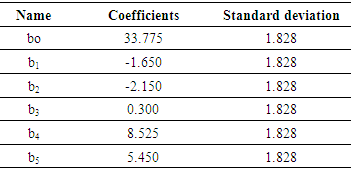The test of coefficients significance of the model has been realized stipulating that a coefficient is significant if its absolute value is superior to twice the standard deviation σ . Parting from this principle, it is the medium coefficient b0 (33.775) and the principal effects b2 (-2.150), b4 (8.520) and b5 (5.450), respectively due to the mass of the absorbent, the pollutant concentration and the middle pH are significantly different from zero. The factors linked to the granulometry (X1) and the contact time (X3) are not significant because their respective coefficients respective b1 (-1.650), b3 (0.3) are inferior to the double of standard deviation.
The Pareto diagram shows the contributions of factors on the ions nitrates removal rate ACT on figure (5).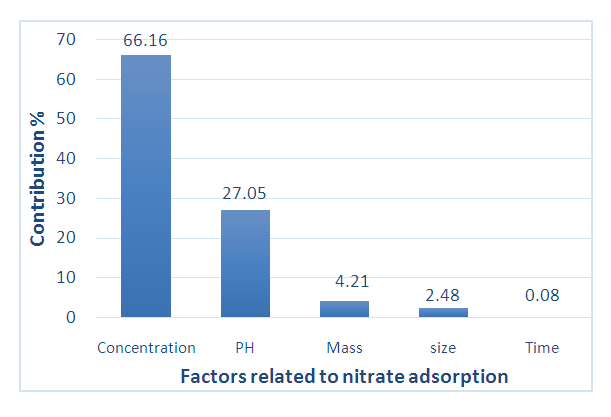Figure 5. Pareto chart showing the contributions of factors in nitrate removal
The diagram shows that the diagram initial concentration is the factor which influences the most the nitrates removal by the corbula trigona seashells. It contributes at the rate of 66.16% in the variability of the studied answer with a positive sign. The increase of the initial concentration of nitrate from 30 to 60 mg. L-1 leads to an increase of the nitrate remaining content in the aqueous medium. In other words, the effectiveness of the treatment decreases with the concentration. The effect of the initial concentration has been proved by certain authors  who demonstrated that the increase of the ions nitrates concentration in the solution permits to increase the residual concentration. This phenomenon would probably be due to the number of available site for the nitrate on the absorbents which would be insufficient in the process of absorbing a great deal of ions nitrates. The second factor which has a powerful influence on is the initial pH with a contribution of 27.05%. The pH is one of the most influential parameters of absorption which can affect the absorption surface, the functional groups and the solution chemical function . The sign of the coefficient is allotted to the pH is positive indicating that the quantity of nitrate in the middle increases as the middle initial increases. The increase of the pH from 2 to 8 contribute to the decrease of the treatment effectiveness. These results are in concordance with the ones other authors reported . Those ones indicate that for a better removal of nitrate, it is important to work with a pH = 2. Those same results have also been obtained by . Those authors reported in their works processed on the protonated chitosan that the nitrate ions absorption capacity was linked to the pH of the solution in the model of 2-7 and reaches its peak at pH = 3-5. The best removal of nitrate into an acidic medium would be linked to the decrease of the negative load at the surface of the absorbent because of the excessive protons into solutions. An environment of positive load area on the adsorbent would favored the nitrate ions adsorption due to the electrostatic attraction.
The effect of the mass is the third factor which has an impact on the removal of nitrates. It has a contribution at a rate of 4.21% on the response variation with a negative effect on the nitrate residual concentration (b2 = -2.150). In terms of the treatment effectiveness, the effect of the absorbent mass is negative. This could be explained by the fact that can increase the accessibility of the seashells sites. This would lead to a betterment of the nitrates removal. A similar study on the nitrates absorption of an aqueous solution by shells  revealed that the absorption of nitrates increase with the increase of the absorbent dose. For the works ongoing, three parameter: the absorbent mass, the pollutant concentration and the middle pH have been retained for the optimization of ions nitrates removal on corbula trigona seashells powder.
##### 3.2.2. Box-Behnken Design (BBD)
In this second part, three retained factors (the mass of the absorbent, the pollutant concentration and the pH) have been considered as the process independent variables. Their individual effects and their interaction effect on the residual concentration (as response) have been studied aid of the Box-Behnken Design. A quadratic polynomial model has been chosen in the process of developing the mathematical relation between the response and the process variables. The experimental and predicted values of nitrate removal effectiveness corresponding to the variables different combinations are displayed in the following table (8).
 Table 8. Experiment Matrix and the Box-Behnken (PBB) Design Results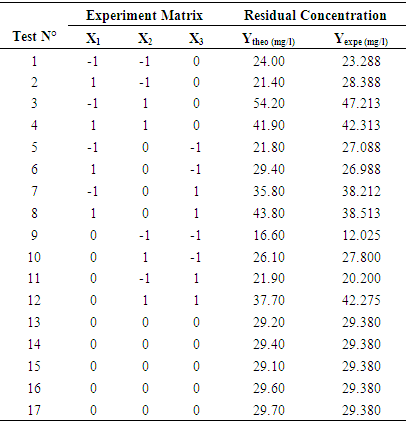By using the multiple regression analysis, the response has been correlated with three studied independent variables, thing which permitted to calculate the model coefficients. Thus, the mathematical relation describing the residual concentration variation can be marked according to encoded variables: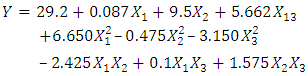(7)
The significancy of the model different coefficients are gathered in table (9). This table reveals that coefficients of the factors (X2) and (X3) are highly significant because of p-values is very weak at the threshold of 5%. Dealing with the factor (X1) coefficient, it is not significant because the p-value is very high. P for the quadratic effects, one obtains the values p > 0.05 then, there is no significance effect for the square of the mass, of the pollutant concentration and the pH. All this denotes that one does not forecast the curve in the responses surface. According to the variance analysis, all the values of the obtained probability by the interactions (X1X2), (X1X3) and (X2X3) are superiors to 0.05, then, there is no significancy effect. In a nutshell, the proposed model fits to the interpretation of the nitrate removal process which would be used in further studies.
 Table 9. Box-Behnken design coefficient estimates and statistics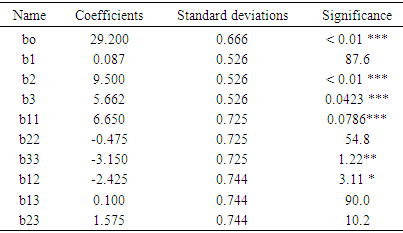The determination of the coefficient values (R2) = 0.84 and of (R2 aj) = 0.64 near 1. This indicates a good correlation between the observed and the predicted values .
##### 3.2.2.1. Analysis of the Variance (ANOVA)
The validity of the models has been tested aid of a variance analysis (ANOVA) of the variables regression of the surface quadratic model of the predicted answer displayed in the table (10). The statistic significancy of all the mode terms has been tested by the F-value and p-value values. The value F is used to check the value of the curve with the residual variance. It implies that the significancy model similarity, and the probability value p > F inferior to 0.05 indicates that the model terms are significant. The value F (61.785) with a weak probability> F (<0.0421) shows that the model terms are significant for the regression model, thing that confirms the validity of the model. . Afterward, one must keep in mind that a model is well adjusted if the sum of the square due to the residues is inferior to the third that is (33.33%) of the squares sum due to the regression . Yet, the table of the variance analysis provided by the Nemrod-W software is that the sum of the squares of the residues is divided into its components which are Validity and Error. The sum of the squares linked to the error is weak (8.86%) compared with the total sum. This means that the obtained model precisely describes the variations of the response. The high values of R2 and R2adj respectively (0.84; 0.64) confirm the pertinence of the model too  and they show that the model can explain a high percentage of the variations occurred within the responses.
 Table 10. Analysis of the Studied Model Variance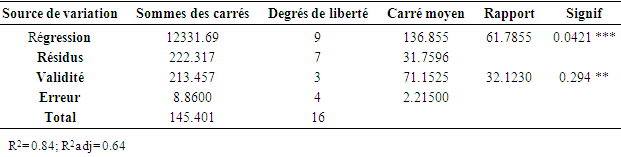##### 3.2.2.2. Validity of the Established Statistic Model
The analysis of the residues has been processed through normal probability, residues compared with the predicted responses. The results are displayed in the figures (6a and 6b). The figure (6a) shows that the residues follows a normal distribution because all the points are closer and form a line . Then, the hypothesis of normality is satisfied. Furthermore, in order to determine the aberrant values, the thresholds of the three standards deviations have been taken into account. One remarks a good distribution of the residues according to the calculated results in figure (6b) . This suggests that the variance of the original observations is constant for all the values of the response. Finally, (6C) indicates that the experimental performances and the estimates are distributed around the line of regression (R2 =0.82) which tends to 1, then, one can conclude that the model is applicable.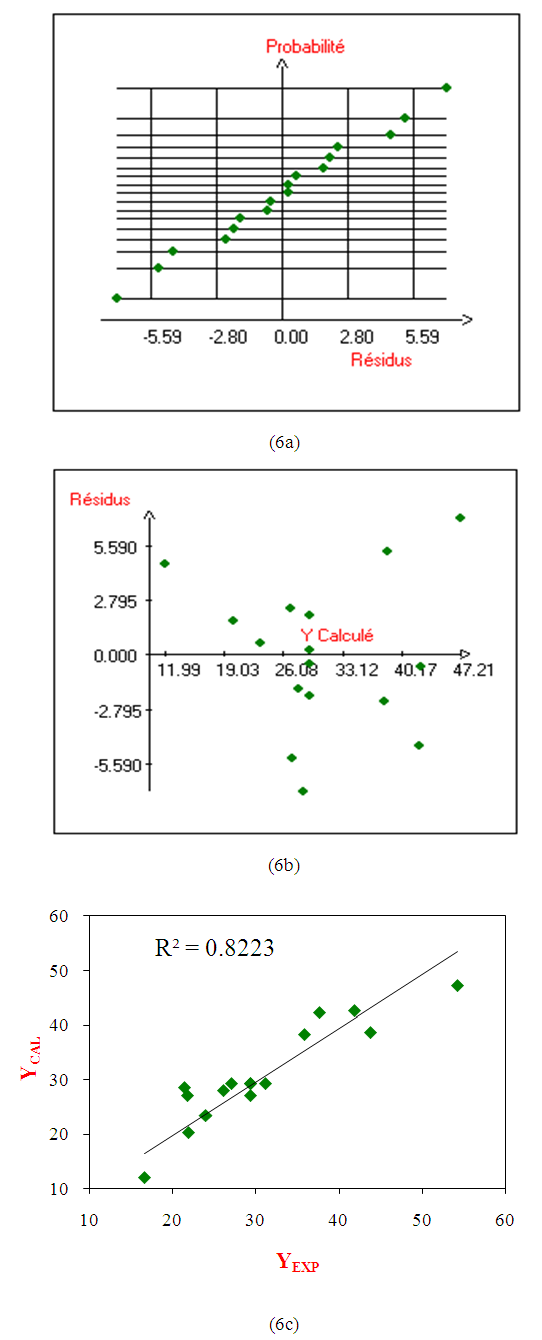Figure 6. Normal Probability (6a), Dispersion of the residues (6b), Distribution of the predicted and experimental data (6c)
##### 3.2.2.3. Analysis of the Surfaces of the Model Responses
The results of the investigated experimental plan on the removal of nitrate aid of corbula trigona seashells permits to obtain the surface of the response and the contour represented on figure (7). This figure shows the effect of the adsorbent mass and its initial concentration, and the pH value settled at center of the domain. This graph presents a maximum of points at the surface of the response. The best rates of the nitrates removal (44.66%) are obtained for weaker values of the concentration (30-45 mg/l) and the increase of the adsorbent mass. The pH of the solution is a parameter which influences the physisco-chemical properties of the solution and the surface of the absorbents favoring the removal of ions nitrates in the solution. It is worth noting that the nitrate ions are negatively charged and their removal is not possible into a basic solution because of the repulsive forces between the materials surfaces and the nitrate ions. However, with some more acidic pH values, the conditions the formation of active species are favorable because these pH better the transfer of ions. Moreover, in an aqueous solution, the carbonized species derived from calcite the presented under three forms: H2CO3; HCO3- and CO32- the proportions of which are determined by the pH of the middle solution. Yet, the carbonates are sufficiently solubilized until to reach a weak alkaline pH . This would explain the tendency of the non-activated seashells to remove the nitrate. The effect of the solution pH could also be explained by the exchange mechanism of ions absorption in which the primordial role would be played by the group of the carbonate corbula trigona seashells which have cations of exchange properties. With weaker pH, the seashells have tendency to absorb protons. It is probable that with a weaker pH, nitrates get transformed in nitric acid which attacks the surface of seashells then a part of ions calcium (Ca2+) gets formed and deactivated the seashells surface . In contrast, the obtained result in this study is inferior to the results of the works of  which have used the achatina-achatina seashells called red snails for waste water processing where the concentration of nitrate has passed from 41.01 to 13.53 mg/l that is 67.03% of the eliminated nitrates. This difference would be due to the modification of the surface of seashells after a chemical activation.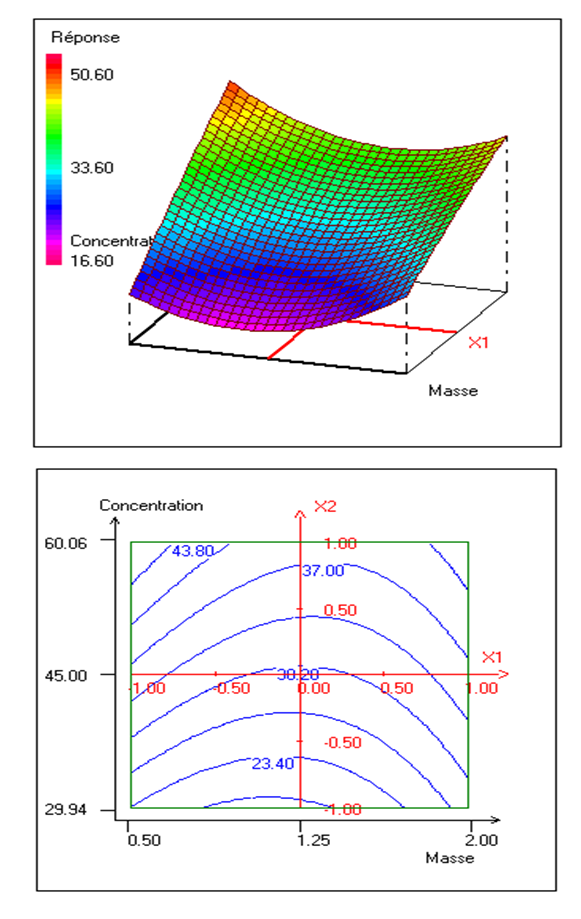Figure 7. Response surface and contour plot showing the evolution of nitrate removal rate as a function of adsorbent mass and pollutant concentration at pH = 5
##### 3.2.2.4. Determination of Optimal Conditions for Nitrate Removal
In the framework of this work, the optimization of the nitrates removal consists in determining the optimum values of the mass, of the nitrates concentration and the initial pH of the solution which permits to maximize the removal of nitrates. The predicted optimum conditions by the consigned models in table 11 have been realized in the conditions of optimum functioning. The elimination of obtained nitrates was of 53.76%. This value is near of the one obtained by the model. This shows a good existing correlation between the predicted and experimental values, then demonstrating the eligibility of the models in their faculty to predict the experimental responses. After examining the optimum conditions proposed by the software of analysis Nemrod-W, the effectiveness of the nitrates removal (44.56%) is translated by a residual concentration of mg/l, which is inferior to the concentration recommended by OMS (50 mg/l). The effectiveness of nitrates removal by a pH= 2 has been reported by other authors [41,42]. These results could be explained by the reduction of the negative load at the surface of the adsorbent because of the excess of protons in the solutions. A site of surface with a positive load on the adsorbent would favor the absorption of nitrate ions due to electrostatic attraction. The removal of nitrates by the Corbula trigona (ACT) adsorbents by using a method of surface has never been a scope of study before.
 Table 11. Optimal conditions for nitrate removal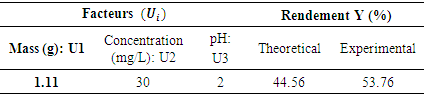### 4. Conclusions

This study was about the investigation of the removal of nitrate ions of waters by adsorption on Corbula trigona seashells (ACT). The characterization of the wastes showed that those ones are majorly constituted of calcite (CaCO3). In this work, the methodology of the experiment plan has been applied for modeling and determining the optimum conditions in the nitrate removal process. On the one hand, the Plackett-Burman Design (PBD) has demonstrated that three factors were influencing (the mass of the adsorbent, the initial concentration of the pollutant and the pH) the percentage of the nitrate ions removal. For the next step, the Box-Behnken Design (PBD) has been used to determine the optimum conditions of nitrate ions removal. The analysis of the variance (ANOVA) of the model has permitted to show that this one is significant, ensuring then a good adjustment of the experimental data. Finally, the optimization of the model presents the following optimum conditions: a mass of 1,11g, an initial concentration of 30mg/l and a pH= 2 for a rate of nitrates removal of more than 53%. These results registered to the scale of laboratory could open some interesting perspective for the practical applications of the waste water processing.